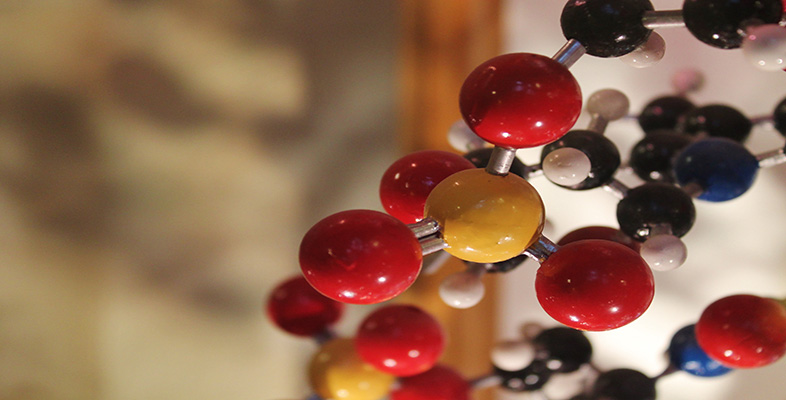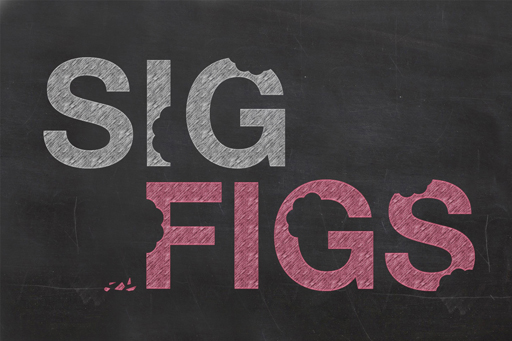Basic science: understanding numbers

Start this free course now. Just create an account and sign in. Enrol and complete the course for a free statement of participation or digital badge if available.

Free course

# 3.3 Can you eat sig figs?Figure _unit3.4.1 Figure 3

The last section discussed rounding to decimal places, one of the ways in which scientists communicate their level of confidence or the precision of a number. In this section you will look at rounding to significant figures. While both approaches are valid, rounding to significant figures is a more common approach in science.

The word significant means having meaning, and the significance of an individual digit within a number depends on its position. For example, the initial weight of the peach presented earlier was 64.375 g. This number has been presented to 5 significant figures. The 6 at the beginning of the number is the most significant figure because it tells you that the number is sixty-something. It then follows that the 4 is the next significant figure, and so on until all the digits are accounted for.

Zeros are also important because their significance varies depending on their position in the number. For example, a raindrop has a volume of 0.034 cm3. Despite the first two zeros, the most significant digit in this number is actually the 3. So here, where zeros are at the start of a number, they are largely ignored. In contrast, following a twenty minute shower, a puddle may have a volume of 108.472 cm3. Here, unsurprisingly, the most significant figure is the 1, however the zero is also a significant figure in this number and its presence doesn’t mean that all the digits to the right can be ignored. The same rules apply for zeros situated at the end of a number and can be used to indicate the confidence in the number. For example, if we really knew the volume of the raindrop to more decimal places, the number could be reported as 0.0340. This would indicate that we are confident of the volume to the fourth number after the decimal point.

You can use the same rounding rules that were introduced previously to round a number to a specified number of significant figures. For example, if you rounded the weights of the three fruits presented in the earlier section to three significant figures, this is what your results would be:

## Table _unit3.4.1 Table 2 The water content of different fruits to three significant figures

Fruit Weight before (g) Weight rounded to 3 sig figs (g)
Watermelon 160.156 160
Cucumber 127.751 128
Peach 64.375 64.4

Can you see how you would come to these figures? While rounding to a number of figures after the decimal produces slightly different results for rounding to sig figs, both are valid and reflect confidence in the result.

You probably round things more often than you realise, most likely when you are shopping. Most people automatically round up (or down) the price of an item so that it is easier to remember or combine with other item prices. However, human perception isn’t always that accurate. You’ve probably seen prices carefully set to appear lower. For example, a new phone or a spectacular pair of shoes can look like a bargain if priced at £199, but don’t be fooled into thinking that it’s meaningfully less than £200.